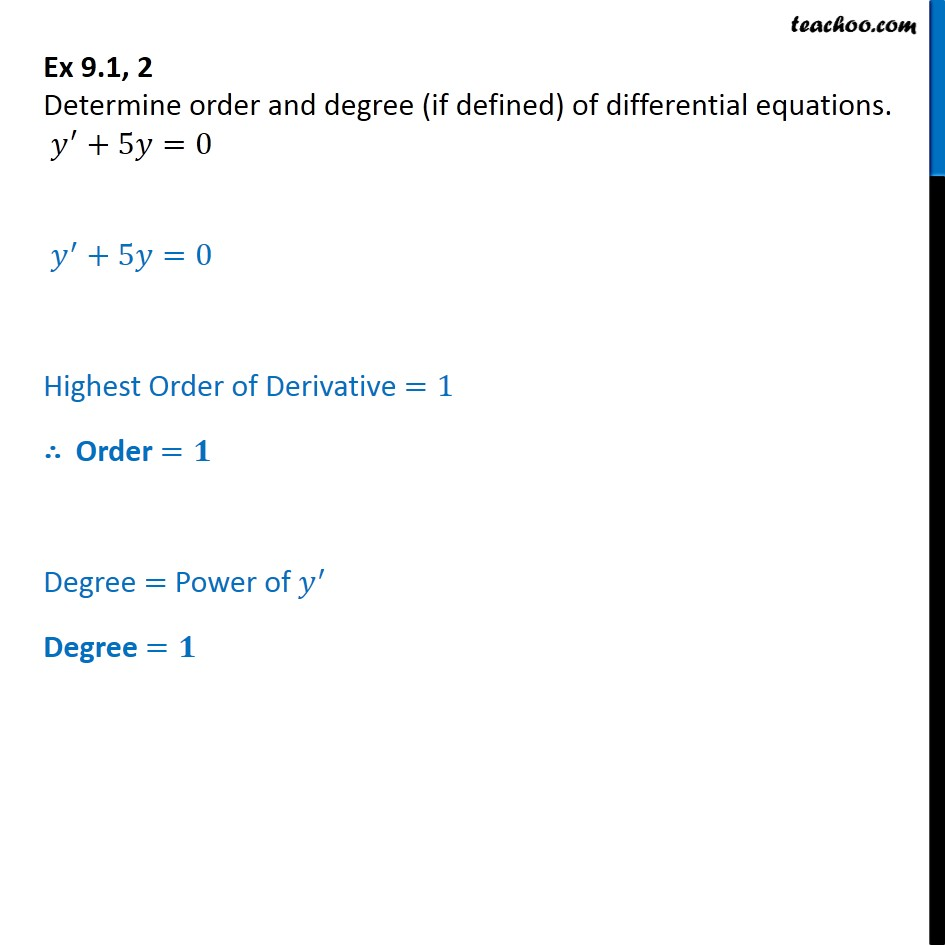Order and Degree

Chapter 9 Class 12 Differential Equations
Concept wiseIntroducing your new favourite teacher - Teachoo Black, at only ₹83 per month

### Transcript

Ex 9.1, 2 - Chapter 9 Class 12 Differential Equations - NCERT Determine order and degree (if defined) of differential equations. y′ + 5𝑦 = 0 Highest Order of Derivative =1 ∴ Order = 1 Degree = Power of y' Degree = 1